## Example Questions

### Example Question #11 : Percentage

Molly needs to buy a new microwave. Two stores, A and B, sell the exact model microwave that Molly needs to buy; however, stores A and B are selling this microwave at two different prices. Store A is selling the microwave for its regular price of $250. Store B is having a 20% off sale on all kitchen appliances. If the regular price of the microwave at store B is$275, how much money will Molly save if she purchases the microwave at store B with the additional 20% discount compared to if she were to purchase the microwave at store A?

$30$25

$50$20

$75 Correct answer:$30

Explanation:

If Molly buys the microwave at store B at the discounted price, we need to calculate what the price of the microwave is after the 20% discount. In order to do so, we must multiply 20% with the $275 regular price of the microwave at store B. 20% is 0.20. So, 0.20 *$275 = $55 This is the discount we will receive. That means that we must subtract$55 from the regular price of $275.$275 – $55 =$220

This is the price of the microwave at store B after the 20% discount.

Now we must compare the prices from store A and B.

Store A sells the microwave for $250. Therefore,$250 – $220 =$30 saved.

### Example Question #12 : Percentage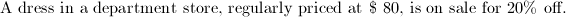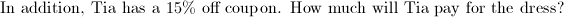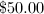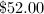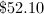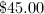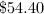Explanation:

Sale price: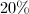off: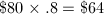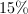coupon: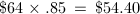Company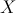is introducing its newest smartphone model, so it is reducing the price of the previous model by 85%.  If the previous model used to sell for $180, what is its new price? Possible Answers: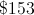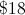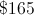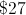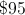Correct answer:Explanation: The sale price is 85% less than the original which is the same as 15% of the original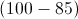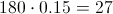### Example Question #14 : Percentage An item is full-priced at$75. It is reduced by 10%, and then you apply a 25% off coupon to that price. There is a 7% sales tax on your purchase. How much do you pay?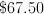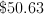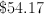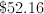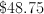Explanation:

The item begins at $75. it is 10% off.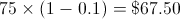You then take an additional 25% off: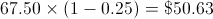There is then a 7% sales tax: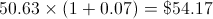### Example Question #15 : Percentage Julie goes shopping at Gap. There is a storewide sale of 30% off. She buys a sweater on clearance that gets an additional 50% off. If the sweater was originally$50, how much did she pay?

$21.50$10

$25$17.50

$35 Correct answer:$17.50

Explanation:

The original price was $50. First you take 30% off (50 * (100 - 30)/100 =$35). Then you take an additional 50% off the new price (35 * 50/100 = 17.50)

### Example Question #11 : How To Find The Sale Price

If Stacy paid $26 for a shirt at a 30%-off sale, what was the original price of the shirt? Possible Answers: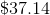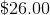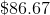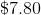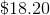Correct answer:Explanation: If the shirt is$26 after 30% was taken off, then cost of the the shirt ($26) is 70% of the original price. We set up an equation that states: 0.7x = 26 (0.7 is the decimal value of 70%) x = 26/0.7 =$37.14

### Example Question #17 : Percentage

A pair of shoes originally sells for $250. There is a sale, and the shoes are then sold for 20% off. The shoes are then marked down an additional 35%. If sales tax is 7%, what can you buy the pair of shoes for today, including tax? Possible Answers:$137.00

$162.50$139.10

$130.00$214.70

$139.10 Explanation: The shoes are first marked down 20%. 20% of$250 = .2 x $250 =$50

Sales price = $250 -$50 = $200 The second markdown is 35%. 35% of$200 = .35 x $200 =$70

New price = $200 -$70 = $130 Calculate the sales tax: 7% of$130 = .07 x $130 =$9.10

Total price = $130 +$9.10 = $139.10 ### Example Question #11 : Percentage Your friend works at a computer store. She can get a 15% discount on any item’s current price for as many items as she wants. She wants to buy a laptop for$2200 and a smartphone for $200. Local sales tax where your friend lives is 8%. What will be her total cost to buy these two items? Possible Answers:$2203.20

$2400$2592

$2040$1848.08

$2203.20 Explanation: First, find the total price of the products$2200 + $200 =$2400.

Then apply the 15% discount: $2400 * 0.15 =$360

Subtract the discount: $2400 –$360 = $2040. Then find cost of sales tax$2040 * 0.08 = 163.20

Add: $2040 + 163.20 =$2203.20.

(An alternative would be to multiply the cost by 1.08.)

### Example Question #11 : How To Find The Sale Price

A person is shopping for clothes and notices that a pair of pants that reguarly costs \$50 are 30% off. This person also has a coupon for 15% off of pants that can be applied after the sale price. What is the final price of the pants that person will pay in dollars? (Assume there is no sales tax)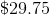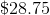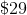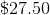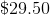Explanation:

First multiply the cost of the pants by the sale price and subtract this value to give the sale price of the pants.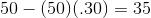Then apply the coupon by multiplying this price by the value of the coupon and subtracting it from the sale price of the pants.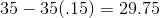Alternatively, start by subtracting the discount from one and multiply by the cost of the pants.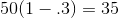Then take the discount of the coupon and subtract it from 1 and multiply by the sale value of the pants.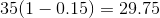### Example Question #12 : How To Find The Sale Price

A customer wants to buy a candy bar that costs fifty cents.  It is taxed at eleven percent by the store.  What's the price that the customer has to pay, rounded to the nearest cent?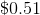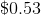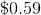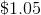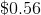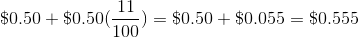The customer will have to payfor the candy bar.